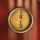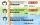# Rational numbers + real numbers - math problems

#### Number of problems found: 6

• Distance of numbersWhich number has the same distance from the numbers -5.65 and 7.25 on the numerical axis?
• FactorsCan the expression ? be factored into rational factors?
• Closest natural numberFind the closest natural number to 4.456 to 44.56 and 445.6.
• ServerCalculate how many average minutes a year is a webserver is unavailable, the availability is 99.99%.
• Discounts on rail (ZSSK)The railway company ZSSK offers a discount of 15% (REGIONAL) for 5 Eur/year. Calculate the real discount rate as a percentage, where passengers will travel 19 Eur per week.
• Youth trackYouth track from Hronská Dúbrava to Banská Štiavnica which announced cancellation attracted considerable media attention and public opposition, has cost 6.3 euro per capita and revenue 13 cents per capita. Calculate the size of subsidies to a trip group o

We apologize, but in this category are not a lot of examples.
Do you have an interesting mathematical word problem that you can't solve it? Submit a math problem, and we can try to solve it.

We will send a solution to your e-mail address. Solved examples are also published here. Please enter the e-mail correctly and check whether you don't have a full mailbox.

Please do not submit problems from current active competitions such as Mathematical Olympiad, correspondence seminars etc...